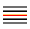Signal Averager is a signal that calculates average values of all past changes from its parent signal.

For example, try to make an Averager window of some existing spectrum window. After the Averager window is created, it contains exactly the same data as the current spectrum in its parent window. Now, start your analysis system and make the spectrum values change. The Averager window collects all changes of the spectrum and calculates their average. This way, you will get the spectrum average through time. You can apply this option to any kind of signal.

After opening Signal tools/Averager menu option ortoolbar button, the following dialog will appear:You can choose between two averaging methods:

• Linear average: new values are calculated as a simple average between all values, i.e. An = (a1 + a2 +a3 + ... +an)/n

• Exponential average: An = (1 - w) * An-1+ w*a  ( w = <weight value in %> / 100.0 )

If you want to reset all Averager values and start with the averaging again, select “Reset data” from its context menu.

If you want to stop or start the averaging process, select the unlink or link option from the main menu or toolbar.

The same function can be used to calculate the average from several signals. You can find more information about it in Control Window Basics